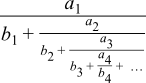Boost C++ Libraries

...one of the most highly regarded and expertly designed C++ library projects in the world.

This is the documentation for an old version of boost. Click here for the latest Boost documentation.

Continued Fraction Evaluation

Synopsis

#include <boost/math/tools/fraction.hpp>

namespace boost{ namespace math{ namespace tools{

template <class Gen, class U>
typename detail::fraction_traits<Gen>::result_type
continued_fraction_b(Gen& g, const U& tolerance, boost::uintmax_t& max_terms)

template <class Gen, class U>
typename detail::fraction_traits<Gen>::result_type
continued_fraction_b(Gen& g, const U& tolerance)

template <class Gen, class U>
typename detail::fraction_traits<Gen>::result_type
continued_fraction_a(Gen& g, const U& tolerance, boost::uintmax_t& max_terms)

template <class Gen, class U>
typename detail::fraction_traits<Gen>::result_type
continued_fraction_a(Gen& g, const U& tolerance)

//
// These interfaces are present for legacy reasons, and are now deprecated:
//
template <class Gen>
typename detail::fraction_traits<Gen>::result_type
continued_fraction_b(Gen& g, int bits);

template <class Gen>
typename detail::fraction_traits<Gen>::result_type
continued_fraction_b(Gen& g, int bits, boost::uintmax_t& max_terms);

template <class Gen>
typename detail::fraction_traits<Gen>::result_type
continued_fraction_a(Gen& g, int bits);

template <class Gen>
typename detail::fraction_traits<Gen>::result_type
continued_fraction_a(Gen& g, int bits, boost::uintmax_t& max_terms);

}}} // namespaces
Description

Continued fractions are a common method of approximation. These functions all evaluate the continued fraction described by the generator type argument. The functions with an "_a" suffix evaluate the fraction:and those with a "_b" suffix evaluate the fraction:This latter form is somewhat more natural in that it corresponds with the usual definition of a continued fraction, but note that the first a value returned by the generator is discarded. Further, often the first a and b values in a continued fraction have different defining equations to the remaining terms, which may make the "_a" suffixed form more appropriate.

The generator type should be a function object which supports the following operations:

Expression

Description

Gen::result_type

The type that is the result of invoking operator(). This can be either an arithmetic type, or a std::pair<> of arithmetic types.

g()

Returns an object of type Gen::result_type.

Each time this operator is called then the next pair of a and b values is returned. Or, if result_type is an arithmetic type, then the next b value is returned and all the a values are assumed to 1.

In all the continued fraction evaluation functions the tolerance parameter is the precision desired in the result, evaluation of the fraction will continue until the last term evaluated leaves the relative error in the result less than tolerance. The deprecated interfaces take a number of digits precision here, internally they just convert this to a tolerance and forward call.

If the optional max_terms parameter is specified then no more than max_terms calls to the generator will be made, and on output, max_terms will be set to actual number of calls made. This facility is particularly useful when profiling a continued fraction for convergence.

Implementation

Internally these algorithms all use the modified Lentz algorithm: refer to Numeric Recipes in C++, W. H. Press et all, chapter 5, (especially 5.2 Evaluation of continued fractions, p 175 - 179) for more information, also Lentz, W.J. 1976, Applied Optics, vol. 15, pp. 668-671.

Examples

The golden ratio phi = 1.618033989... can be computed from the simplest continued fraction of all:We begin by defining a generator function:

template <class T>
struct golden_ratio_fraction
{
typedef T result_type;

result_type operator()
{
return 1;
}
};

The golden ratio can then be computed to double precision using:

continued_fraction_a(
golden_ratio_fraction<double>(),
std::numeric_limits<double>::epsilon());

It's more usual though to have to define both the a's and the b's when evaluating special functions by continued fractions, for example the tan function is defined by:So its generator object would look like:

template <class T>
struct tan_fraction
{
private:
T a, b;
public:
tan_fraction(T v)
: a(-v*v), b(-1)
{}

typedef std::pair<T,T> result_type;

std::pair<T,T> operator()()
{
b += 2;
return std::make_pair(a, b);
}
};

Notice that if the continuant is subtracted from the b terms, as is the case here, then all the a terms returned by the generator will be negative. The tangent function can now be evaluated using:

template <class T>
T tan(T a)
{
tan_fraction<T> fract(a);
return a / continued_fraction_b(fract, std::numeric_limits<T>::epsilon());
}

Notice that this time we're using the "_b" suffixed version to evaluate the fraction: we're removing the leading a term during fraction evaluation as it's different from all the others.